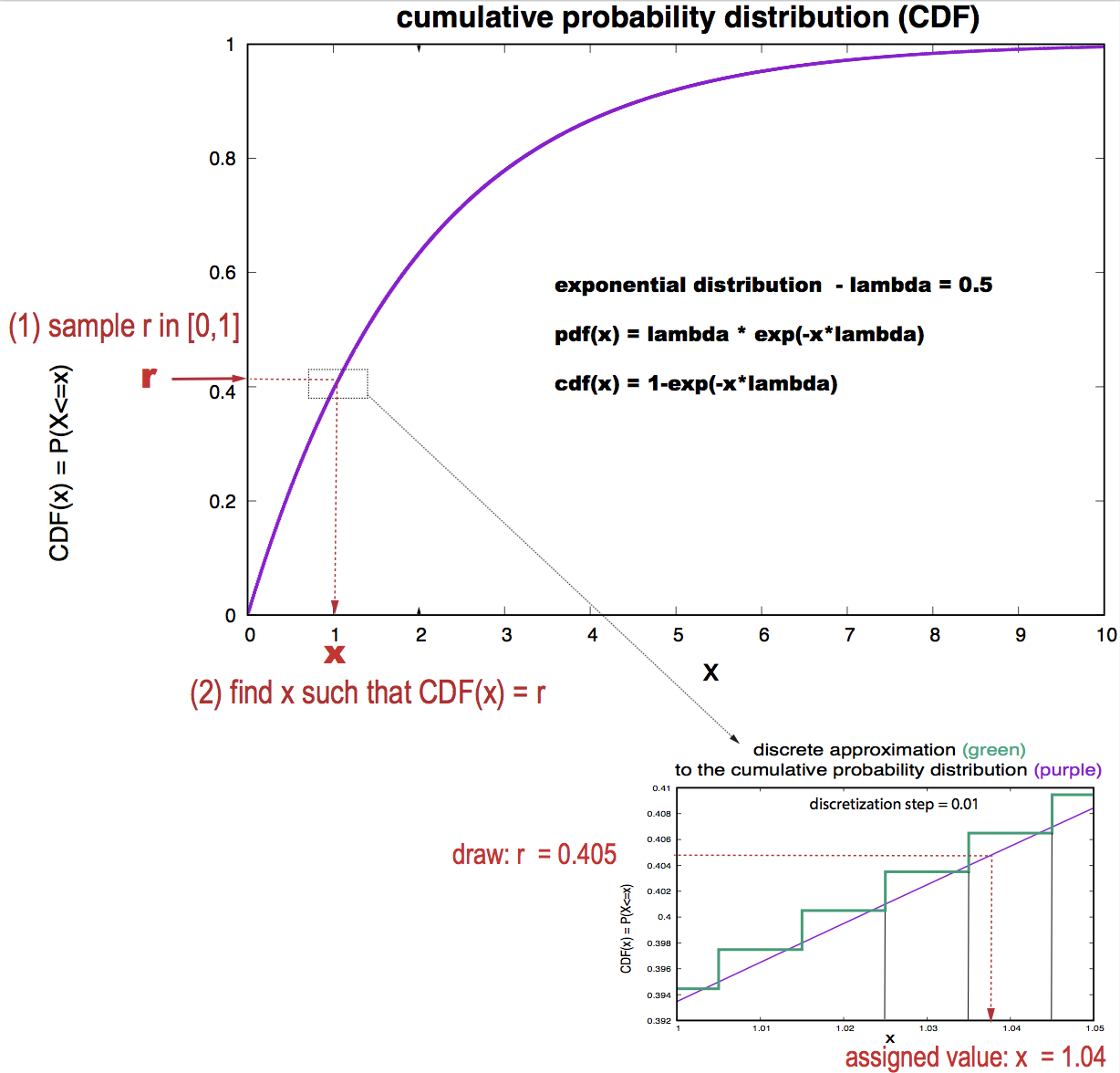# week 01:

The purpose of our homework this week is to develop intuition for measurements resulting from different probability distributions. When values are recorded that obey some fundamental distribution, we want an idea of what they will look like. We would also like to have a sense of how many samples it takes to identify the underlying distribution with some confidence.

## Sampling from a distribution

Sampling from the distribution is similar to randomly throwing a dart at the distribution, and recording the x-coordinate of where it struck. For example, with the Gaussian distribution, we can expect to find the darts strike most frequently near the average value (the center of the distribution). Meanwhile, darts hit the uniform distribution evenly at all x-coordinates.

How can we achieve this sort of sampling with a simple computer script? Conveniently you can directly sample from distributions with built in scripts such as: rand, exprnd(mu), normrnd(mu,sigma).

However, the goal here is that you do it yourself just using only the rand() function, so you understand what is going on, and pay attention to the implementation details.

### Algorithm for discrete sampling using only the rand() function

So we said that sampling a probability distribution is like throwing darts that land randomly somewhere under its curve. How can we accomplish this if we can only “throw darts” uniformly between 0 and 1?

Consider the exponential distribution, we are much more likely to hit a dart close to the value 0 than some value much past our mean. The $x$ values corresponding to higher probability values span more space for our dart to land, so they are over-represented. This translates directly to the cumulative distribution function (exponential CDF: second figure on the right https://en.wikipedia.org/wiki/Exponential_distribution).Figue 1. Sampling algorithm using only the rand() function. In purple, the exponential distribution for lambda = 0.5. In green, the discrete approximation using a window of size 0.01.

In general, for a probability density function (PDF) $p(x)$, defined in a range $[x_{min},x_{max}]$, the cumulative distribution function (CDF) $P(x)$ is given by

\begin{equation} P(x) = \int_{x_{\min}}^x p(y) dy \end{equation}

is a function that ranges over the interval [0,1], since it is a probability.

The simplest way is to construct the CDF numerically by discretizing the space $[x_{min},x_{max}]$ as,

\begin{equation} x_i = x_{min} + \Delta\,i \quad \mbox{in the range}\quad 1\leq i \leq N, \end{equation}

for some small interval value $\Delta$, such that $x_{max} = x_{min} + \Delta\,N$.

The discrete form of the CDF $P$ is given by the $N$ dimensional vector \begin{equation} \mbox{cdf}[i]\sim\sum_{j=1}^i p(x_{j})\, \Delta = \mbox{cdf}[i-1] + p(x_i)\,\Delta, \end{equation}

as described in the insert of Figure 1.

The sampling algorithm is:

1) Generate a random number $r$ between 0 and 1

2) Find the index $i$ such that

\begin{equation} \mbox{cdf}[i] \leq r < \mbox{cdf}[i+\Delta]. \end{equation}

Then the value sampled from the distribution is

\begin{equation} x = x_{min} + i\,\Delta. \end{equation}

That is, $x$ is the highest value such that $P(x) \leq r$. See Figure 1.

## The entropy of a continuous distribution can be positive or negative

In class, we introduced the entropy of a distribution

\begin{equation} \mbox{Entropy}(X) = H(X) = \sum_i p_i \log \frac{1}{p_i}. \end{equation}

I mentioned that the entropy of a distribution was always positive, and could only be zero if one of the elements $i$ had probability $p_i=1$.

I was following Shannon (bottom of page 11), but I failed to realize that Shannon’s statement applies only to discrete distributions.

### The Shannon entropy of the exponential distribution can be negative

In fact, when you did your homework, you should have noticed, that, for the exponential distribution, given by the probability density function

\begin{equation} p(x) = \frac{1}{\mu}\exp^{-\frac{x}{\mu}} \end{equation}

its entropy is given by

\begin{equation} 1 + \log(\mu), \end{equation}

and it can take negative values for many values of the mean $\mu$!.

Robert B. Ash in his 1965 paper Information Theory (page 237) noted this: unlike a discrete distribution, for a continuous distribution, the entropy can be positive or negative, in fact it may even be $+\infty$ or $-\infty$.

The reason is that for a discrete distribution, the normalization condition

\begin{equation} \sum_i p_i = 1 \end{equation}

requires that no individual probability can be larger than one, that is, $0\leq p_i \leq 1$ for all possible values of $i$.

In contrast, for a continuous distribution, the normalization condition

\begin{equation} \int p(x)\, dx = 1 \end{equation}

does not require for all probability density values, $p(x)$, to be smaller than one. For instance if you look at the wikipedia definition of the exponential distribution you can see for $\lambda = 1.5$ that some values of the probability density function are indeed larger than one.

If we discretize the continuous distribution

\begin{equation} \int p(x)\, dx \sim \sum_i p(x_i)\, \Delta = 1 \end{equation}

we see that only the product $p(x_i) \Delta$ for each term $i$ in the sum has to be smaller than 1.

Having values of $p(x)$ that can be larger than one is the cause why the entropy of a continuous distribution

\begin{equation} H = \int p(x)\, \log \frac{1}{p(x)}\, dx. \end{equation}

cannot be guarantee to be positive, as the term $\log \frac{1}{p(x)}$ takes a negative value if $p(x)> 1$.

That means that for a continuous distribution the meaningful quantity to consider is the relative entropy (or Kullback-Leibler distance) relative to some arbitrary reference distribution $p_0$. For any continuous distribution $p(x)$ the relative entropy

\begin{equation} D_{KL}(p||p_0) = \int p(x)\, \log \frac{p(x)}{p_0(x)}\, dx, \end{equation}

is guaranteed to be always positive.